# Inductor

An inductor is an electrical component usually made of a coil of conducting wire that stores energy in a magnetic field. Inductors have an inductance, which is measured in Henry (H). Inductance is defined as the ratio of the voltage to the rate of change of current.

### Air core inductor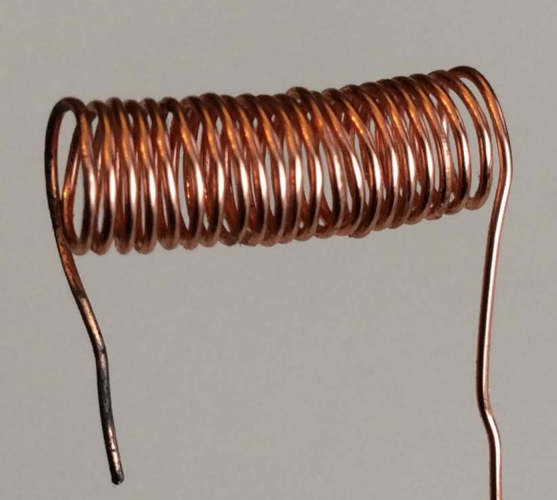Air core inductor
Air core inductor

Air core inductors that consist of a coil of conducting wire with no core. The following formulas are used to calculate the inductance and wire length: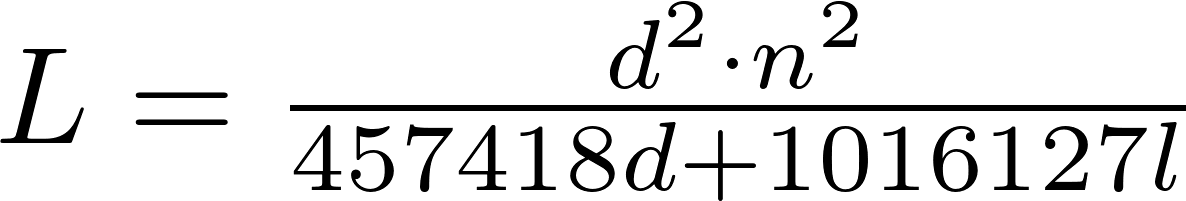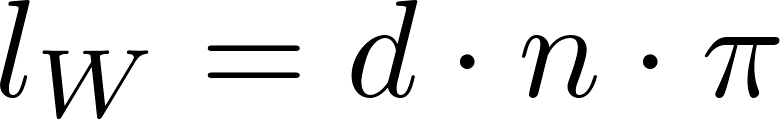### Inductors in series

Inductors in series

Inductors can be connected in series to increase the total inductance. The total inductance is equal to the sum of all individual inductances.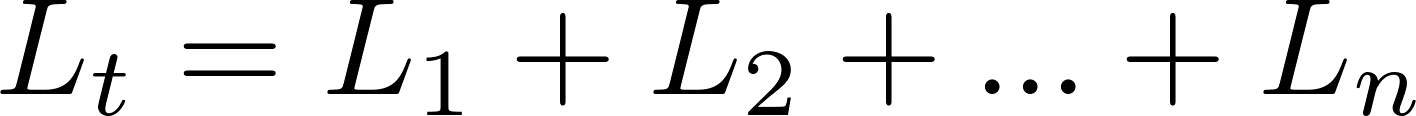### Inductors in parallel

Inductors in parallel

Inductors can be connected in parallel to decrease to total inductance. The total inductance is equal to the reciprocal (inverse) of the sum of the reciprocal of all individual inductances.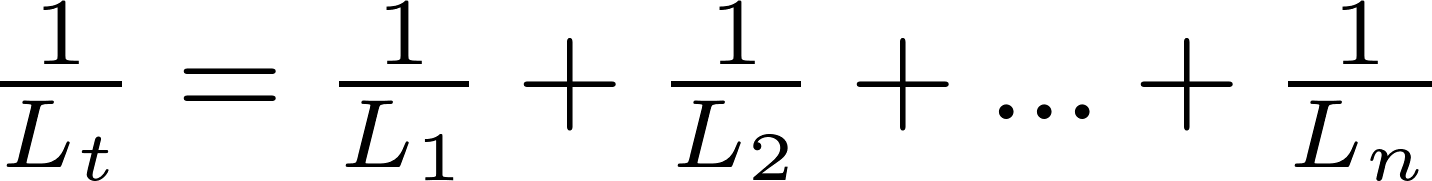### Markings

#### Inductor color code

Inductor color code

Larger inductors use colored bands to indicate the inductance. There are two types of these inductors: inductors with four bands, and with five bands. The first two bands represent a number between 00 and 99. This number is multiplied to ten to the power of the third band to calculate the inductance in microhenry (μH). The fourth band represents the tolerance of the inductor. The fifth band is a silver band at the beginning, which is a military identifier. The five band inductors work the same as their four band counterparts.

color digit multiplication tolerance
black 0 1 -
brown 1 10 1%
red 2 100 2%
orange 3 1000 3%
yellow 4 10,000 4%
green 5 100,000 0.5%
blue 6 1,000,000 0.25%
violet 7 10,000,000 0.1%
grey 8 100,000,000 0.05%
white 9 1,000,000,000 -
gold - 0.1 5%
silver - 0.01 10%

#### Numberic code

Inductor codes

Smaller inductors use a number code to indicate the inductance. The inductance in microhenry (μH) is equal to the first two digits multiplied by ten to the power of the third digit. Some codes have an additional letter at the end, which indicates the tolerance.

letter tolerance letter tolerance
A 0.05nH H 3%
B 0.1nH J 5%
C 0.25nH K 10%
D 0.5nH L 15%
E 0.5% M 20%
F 1% V 25%
G 2% N 30%
Z +80% -20% - -